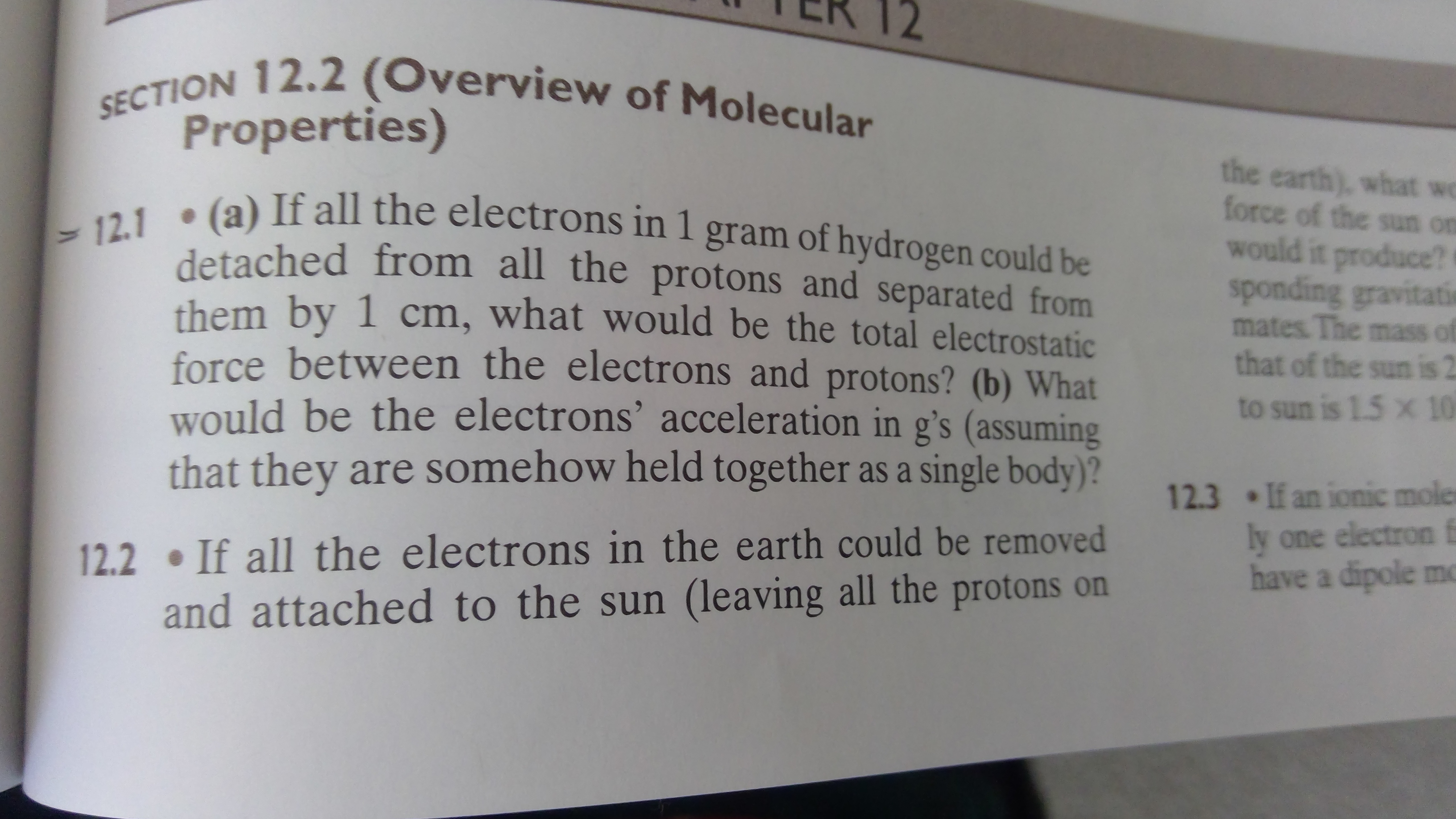# SECTION 12.2 (Overview of MolecularProperties)the earth), what weforce of the sun onwould it produce?sponding gravitatimates. The mass ofthat of the sun is 212.1 (a) If all the electrons in 1 gram of hydrogen could bedetached from all the protons and separated fromthem by 1 cm, what would be the total electrostaticforce between the electrons and protons? (b) Whatwould be the electrons' acceleration in g's (assumingthat they are somehow held together as a single body)?to sun is 1.5 x 1012.3 If an ionic molerly one electron fhave a dipole mo12.2 If all the electrons in the earth could be removedand attached to the sun (leaving all the protons on

Question
13 views

How do I solve for Problem 12.1? This problem is in a chapter called "Molecules," and it is part of a portion called Systems with two or more Atoms.help_outlineImage TranscriptioncloseSECTION 12.2 (Overview of Molecular Properties) the earth), what we force of the sun on would it produce? sponding gravitati mates. The mass of that of the sun is 2 12.1 (a) If all the electrons in 1 gram of hydrogen could be detached from all the protons and separated from them by 1 cm, what would be the total electrostatic force between the electrons and protons? (b) What would be the electrons' acceleration in g's (assuming that they are somehow held together as a single body)? to sun is 1.5 x 10 12.3 If an ionic moler ly one electron f have a dipole mo 12.2 If all the electrons in the earth could be removed and attached to the sun (leaving all the protons on fullscreen
check_circle

Step 1

Calculating the number of electrons and number of protons in 1 g of hydrogen:

Step 2

Calculating the force between all ...

### Want to see the full answer?

See Solution

#### Want to see this answer and more?

Solutions are written by subject experts who are available 24/7. Questions are typically answered within 1 hour.*

See Solution
*Response times may vary by subject and question.
Tagged in

### Science